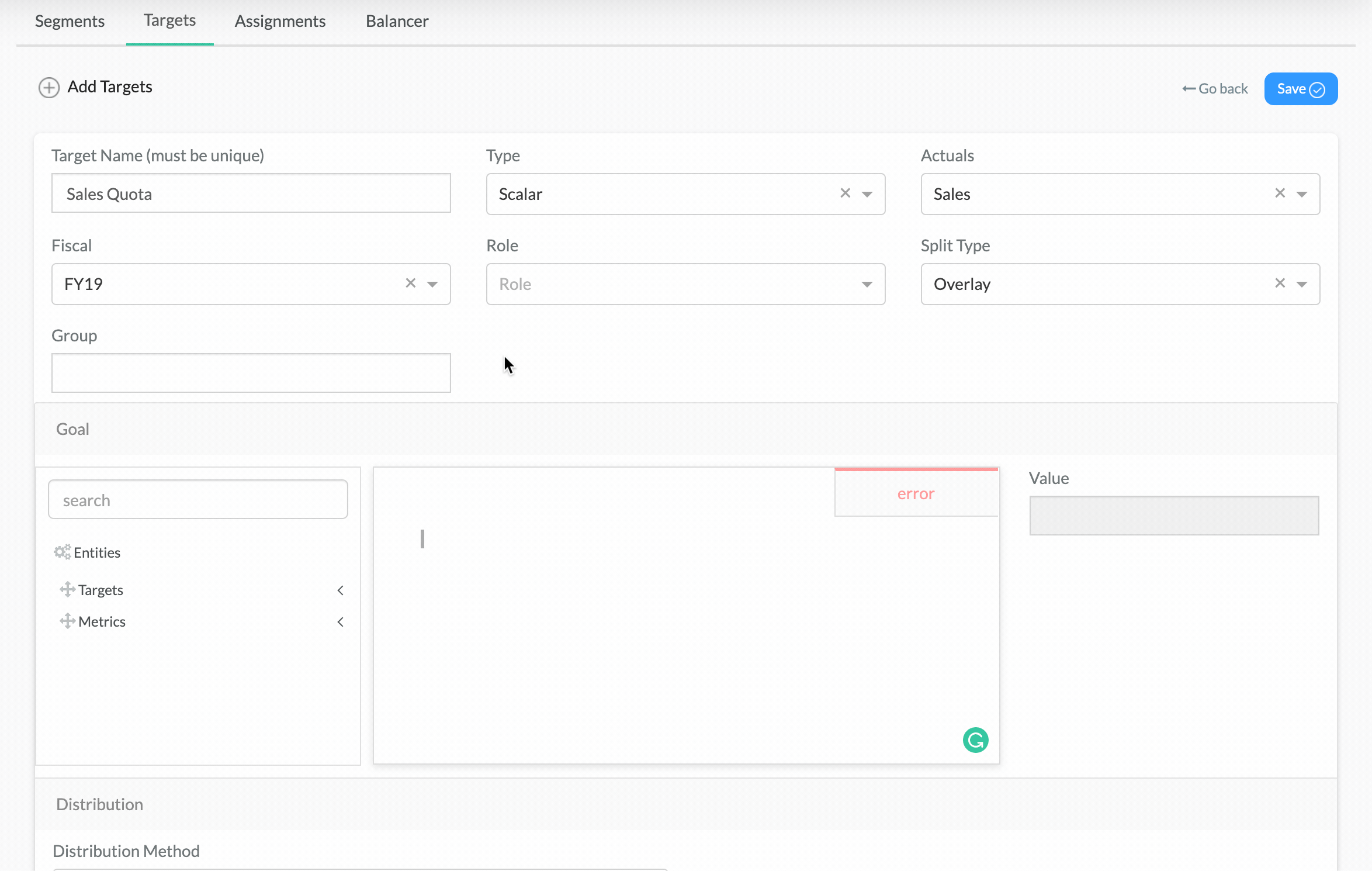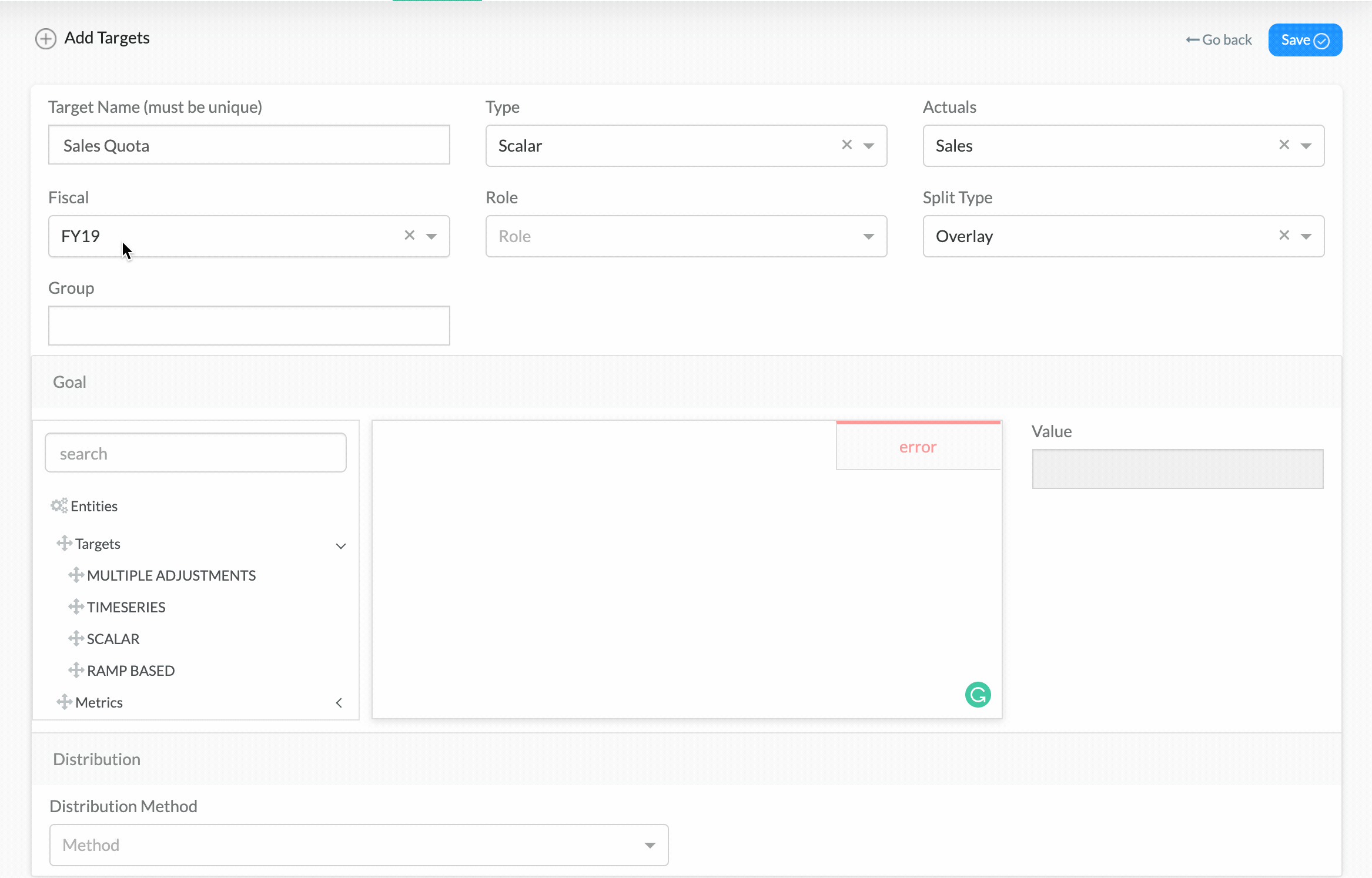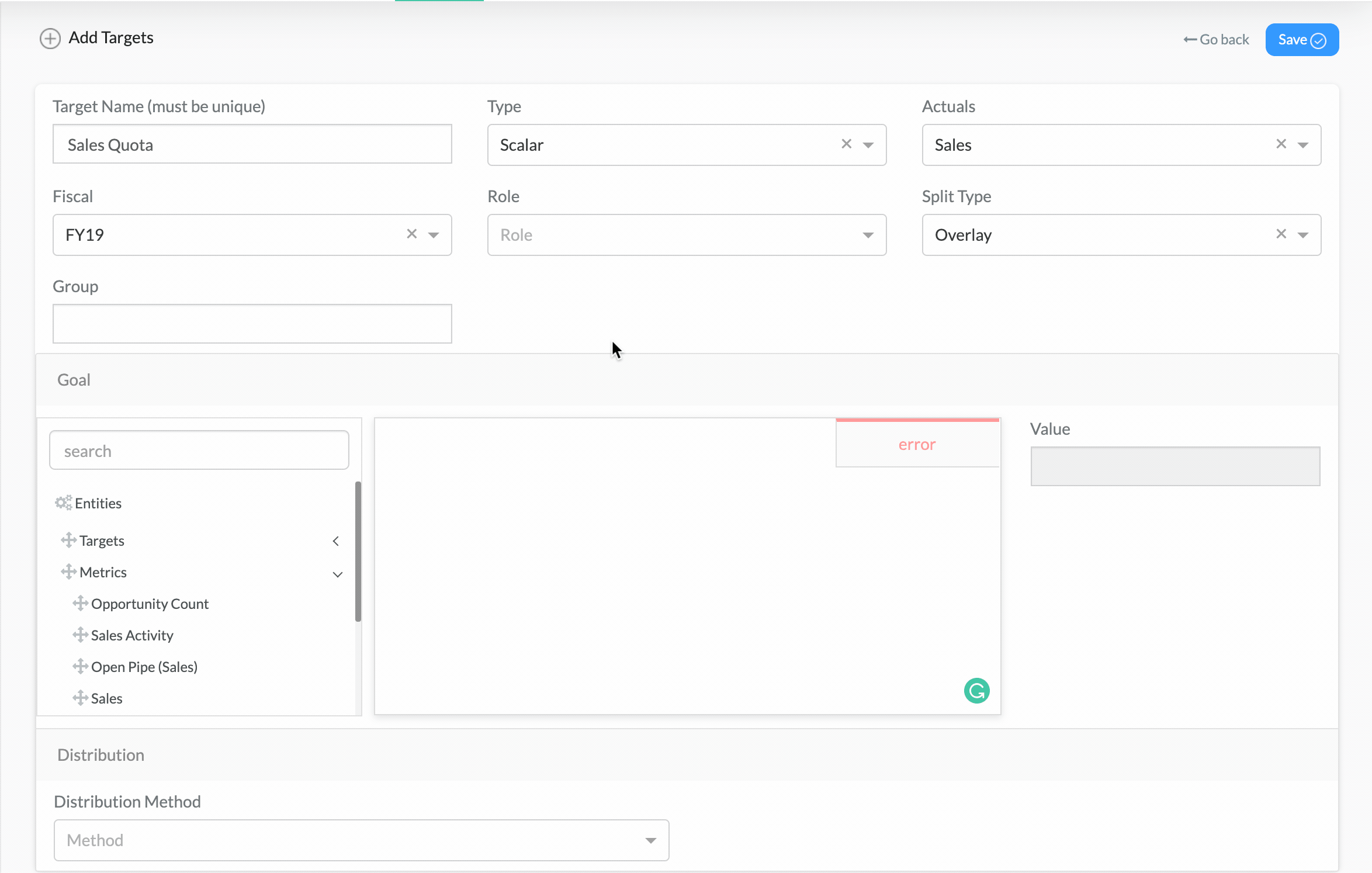# Formula Based Targets

The formula-based goal is a way to construct a goal based on other related variables.

### Various formula variables that are available for use are described below:

1. Constants – static values entered as a number, typically used for assumption constants (e.g., market share, growth %, etc.)2. Other Targets – You can use another target as the basis to create a new target.3. Metrics – Use any metric and the value of that metric for a particular time period and node. For example, use last year’s revenue (i.e. Revenue metric in FY16) for the SMB node and divide it by 4.Using the above variables, predefined functions, and constant values, you can construct a mathematical formula that will generate the amount that will become the goal to be used.